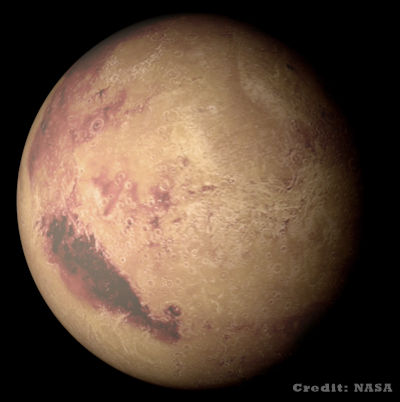Exam-Style Questions on Standard Form

Problems on Standard Form adapted from questions set in previous Mathematics exams.

1.

GCSE Higher

(a) Write 0.0000657 in standard form.

(b) Write 2.371 × 104 as an ordinary number.

2.

GCSE Higher

The number 1.25 × 10n is a cube number.

Find the smallest possible integer value of n.

3.

GCSE Higher

The following table shows the distance from the sun and mass of the eight planets.

Planet Distance from the Sun (km) Mass (tonnes)
Mercury 5.79×107 3.3×1020
Venus 1.08×108 4.87×1021
Earth 1.5×108 5.98×1021
Mars 2.28×108 6.42×1020
Jupiter 7.78×108 1.9×1024
Saturn 1.43×109 5.69×1023
Uranus 2.87×109 8.68×1022
Neptune 4.5×109 1.02×1023

(a) Which planet has the second smallest mass?

(b) Find the difference between the mass of Saturn and the mass of Jupiter.

(c) It is claimed that Saturn is about ten times further from the sun than the Earth. By showing your working explain why you either agree or disagree with this statement.

4.

GCSE Higher

At a particular time the distance between Earth and Mercury was $$7.7 \times 10^7$$ km.

A signal was sent from Earth to Mercury travelling at an estimated speed of $$3 \times 10^5$$ km per second.

(a) how long did the signal take to get to Mercury?

The speed of the signal sent from Earth to Mercury was actually less than $$3 \times 10^5$$ km per second.

5.

IB Studies

The Moon appears to orbit around the Earth with an average radius of 385 thousand kilometres.

(a) Write down this radius, in kilometres, in the form $$a\times 10^k$$, where $$1 \le a\lt 10,k\in \mathbb Z$$

(b) Calculate the distance, in kilometres, the Moon travels around the Earth in one orbit, assuming that the orbit is a circle.

It takes approximately 27.3 days for the Moon to complete one orbit.

(c) Calculate the total distance the Moon appears to travel around the earth during a leap year.

6.

GCSE Higher

The volume of the Moon is $$2.2 \times 10^{10}$$ km3

The volume of the Saturn is $$8.3 \times 10^{14}$$ km3

How many times larger is the radius of Saturn than the radius of the Moon?

Assume that Saturn and the Moon are both spheres and that the volume $$v$$ of a sphere with radius $$r$$ is given by the following formula.

$$v=\frac43\pi r^3$$

7.

GCSE Higher

The surface gravity, $$g$$, of a planet is the gravitational acceleration experienced at its surface. The following formula can be used to find how a planet's gravity compares to Earth's.

$$g=\frac{6.67 \times 10^{-11} \times m}{r^2}$$

Where $$m$$ is the mass of the planet and $$r$$ is the radius.

Find the ratio of the surface gravity on Jupiter and the surface gravity Mars. Give your answer in the form $$n:1$$.

 Mass (kg) Radius (m) Jupiter 1.90 × 1027 6.68 × 107 Mars 6.39 × 1023 3.39 × 106

8.

IB Analysis and Approaches

NASA’s Transiting Exoplanet Survey Satellite (TESS) has discovered a planet between the sizes of Mars and Earth orbiting a bright, cool, nearby star. The planet, called L 98-59b, marks the smallest found by TESS yet. The radius of L 98-59b is $$5.42\times 10^6$$ m.(a) Write down the diameter of the planet in kilometers.

The volume of the planet in cubic kilometers can be expressed in the form $$n\times 10^k$$, where $$1 \le n\lt 10,k\in \mathbb Z$$

(b) Find the value of $$n$$ and the value of $$k$$.

If you would like space on the right of the question to write out the solution try this Thinning Feature. It will collapse the text into the left half of your screen but large diagrams will remain unchanged.

The exam-style questions appearing on this site are based on those set in previous examinations (or sample assessment papers for future examinations) by the major examination boards. The wording, diagrams and figures used in these questions have been changed from the originals so that students can have fresh, relevant problem solving practice even if they have previously worked through the related exam paper.

The solutions to the questions on this website are only available to those who have a Transum Subscription.

Exam-Style Questions Main Page

Search for exam-style questions containing a particular word or phrase:

To search the entire Transum website use the search box in the grey area below.